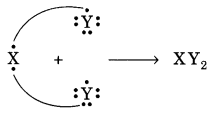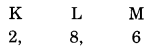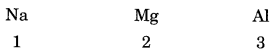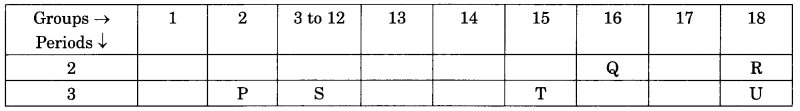# CBSE Solutions for Class 10 Chemistry

#### Select CBSE Solutions for class 10 Subject & Chapters Wise :

What is the difference between the subsequent two reactions ?
(a) Mg + 2HCl ——–> MgCl2 + H2
(b) NaOH + HCl ——–> NaCl + H2O.

Answer :

(a) It is a single displacement reaction,
(b) it's a double displacement also called neutralization .

Which of the subsequent may be a combination reaction and which may be a displacement ?
(a) Cl2 + 2KI ——–> 2KCl + I2
(b) 2K + Cl2 ——–> 2KCl.

Answer :

(a) It is a displacement reaction,
(b) It is a combination reaction.

Identify the foremost reactive and least reactive metal : Al, K, Ca, Au.

Answer :

Most reactive metal : K (potassium) ; Least reactive metal : Au (gold).

Fill in the Blanks : - The addition of oxygen to a substance is called …………. whereas removal of oxygen is called …………. .

Answer :

oxidation, reduction

Fill in the blanks :- ………….. is burnt in air to form magnesium oxide.

Answer :

Magnesium

Fill in the blanks :- Complete the missing components/variables given as x and y in the following reactions:
(a) Pb (NO3)2 (aq) + 2KI (aq) → Pbl2 (x) + 2KNO3 (y)
(b) Cu (s) + 2AgNO3 (aq) → Cu (NO3)2 (aq) + x (s)

Answer :

(a) x → (s); y → (aq);
(b) x → 2Ag

Fill in the blanks :- Na2SO4 + BaCl2 → …………. + 2NaCl

Answer :

BaSO4

Fill in the blanks :- 2 FeSO4 → Fe2O3 + SO2 + ………….

Answer :

SO3

Fill in the blanks :- …………. is the process in which metals are eaten up gradually by the action of air, moisture or a chemical on their surface.

Answer :

Corrosion

Fill in the blanks :- Two antioxidants which are usually added to fat and oil containing foods to prevent rancidity, are …………., …………. .

Answer :

BHA & BHT

Fill in the blanks :- Reaction in which an element displaces another element from its compound is called …………. .

Answer :

displacement reaction

Fill in the blanks :- Reactions in which energy is given out are known as …………. .

Answer :

exothermic reactions

Fill in the blanks :- Precipitation reactions produce insoluble …………. .

Answer :

salts

Fill in the blanks :- The addition of hydrogen to a substance is called …………. whereas removal of hydrogen is called …………. .

Answer :

reduction, oxidation

Fill in the blanks :-  Magnesium is able to displace copper from copper sulphate solution because magnesium is ………….. reactive than copper.

Answer :

more

Fill in the blanks :-  The oxidation and reduction reaction are also called ………….. reactions.

Answer :

redox

Fill in the blanks :-  ………….. can be retarded by storing foods away from light.

Answer :

Rancidity

Fill in the blanks :-  In rusting of iron, reddish brown coating formed on iron is …………..

Answer :

Fe2O3

Fill in the blanks :-  ………….. are added to foods containing fats and oil.

Answer :

Antioxidants

Oxidation is the gain of ………….. or loss of …………..

Answer :

Answer: oxygen, hydrogen

Fill in the blanks :-  Any reaction that produces a ………….. is called a precipitation reaction.

Answer :

Precipitate

Fill in the blanks :-  Zinc and lead are more reactive elements than …………..

Answer :

copper

Fill in the blanks :-  Respiration is an ………….. reaction.

Answer :

exothermic

Why doesn't a wall immediately acquire a white colour when a coating of calcium hydroxide is applied on it?

Answer :

Slaked lime as such is not very white. When applied on the wall, CO2 gas present in air reacts with calcium hydroxide to form calcium carbonate. It is quite white and therefore, imparts white look to the wall.
Ca(OH)2 + CO2(g) ———> CaCO3(s) + H2O(l).

What is rust ?

Answer :

It is a brown mass referred to as hydrated oxide . Its formula is Fe2O3.xH2O.

Two elements X and Y have atomic number 12 and 17 respectively.
(i) Write the electronic configuration of both.
(ii) Which type of bond will they form?
(iii) Write the formula of the compound formed by their combination (in terms of X and Y).

Answer :

(i) The electronic configuration of element X (Z = 12) = 2, 8, 2
The electronic configuration of element Y (Z = 17) = 2, 8, 7

(ii) The bond formed will be of ionic nature. One atom of X will transfer 1 electron each to two atoms of Y.

(iii) The formula of the compound is:The electronic configuration of an element X is:(i) What is the group number of element X in the periodic table?
(ii) What is the period number of element X in the periodic table?
(iii) What is the number of valence electrons in an atom of X?
(iv) What is the valency of X?

Answer :

(i) From the above given electronic configuration we find that element X has 6 valence electrons in the outermost shell), so the group number of element X in the periodic table is 6 + 10 = 16.

(ii) Element X has 3 electron shells (K, L and M) in its atom, so the period number of X is 3. That is, X belongs to 3rd period of the periodic table.

(iii) Element X has 6 valence electrons.

(iv) Element X has 6 valence electrons so it needs 2 more electrons to complete its octet (8 electrons in valence shell) and become stable. Thus, the valency of element X is 2.

How many groups and periods are there in the modern periodic table? How do the atomic size and metallic character of elements vary as we move: (a) down a group and (b) from left to right in a period.

Answer :

Number of groups is 18 and number of periods in the modern periodic table is 7.
(a) All the elements in a group have the same valency. On going down in a group, the atomic size increases because a new shell of electrons is added to the atoms at every step.

(b) On moving from left to right in the periodic table, the valency of the elements first increases from 1 to 4 and then decreases to zero. On moving from left to right in a period, the metallic character of elements decrease because on moving from left to right in a period, the electropositive character of elements decrease.

Na, Mg and Al are the elements of the same period of Modern Periodic Table having one, two and three valence electrons respectively. Which of these elements (i) has the largest atomic radius, (ii) is least reactive? Justify your answer stating reason for each case.   [Delhi 2015]

Answer :(1) Na has the largest atomic radius because on moving from left to right in the periodic table, the atomic radius decreases due to increase in positive charge on the nucleus which pulls the outermost electrons more close to the nucleus and the size of atom decreases.

(2) Al is least reactive because on moving from left to right in the periodic table the nuclear charge increases and the valence electrons are pulled in more close to the nucleus. Therefore, the tendency to lose electrons decreases and hence reactivity decreases.

Write two reasons responsible for the late discovery of noble gases

Answer :

1. Noble gas elements were not present in earth crust as minerals like other elements and were present in air to a very small extent.
2. Their atoms have stable electronic configuration of their outermost shells also called valence shells. (2 in case of He and 8 for other elements). They do not combine with atoms of other elements.

That is why, noble gas elements were discovered at a later stage.

Explain why:
(i) All the elements in a group have similar chemical properties.
(ii) All the elements in a period have different chemical properties.

Answer :

(i) All the elements in a particular group have similar outer shell electronic configuration. Since chemical properties of an element are determined mainly by the outer shell configuration, all the elements in a group have similar chemical properties.

(ii) All the elements in a period have different valence shell electronic configuration because they have different number of electrons in the valence shell. Hence, the elements in a period have different chemical properties.

How does the electronic configuration of an atom of an element relate to its position in the modem periodic table? Explain with one example.

Answer :

The electronic configuration of an atom of an element gives its position in the modern periodic table.
(i) The ‘period number’ of an element is equal to the number of electron shells in its atom.
(ii)

• The group number of an element having upto two valence electrons is equal to the number of valence electrons.
• The group number of an element having more than 2 valence electrons is equal to the number of valence electrons plus 10.

Example: If the electronic configuration of an element is 2, 8, 7.
Then its period number is 3 as it has three electrons shells.
Its group number is 17 as it has 7 valence electrons. (∵ Group no. = 7 + 10 = 17)

Explain clearly, why atomic number of an element is most important to the chemist than its relative atomic mass.

Answer :

Atomic number corresponds to the number of electron in an atom or it reflects the electronic configuration of the element. The elements having similar electronic configurations can then be placed together in the same group, it helps in the systematic classification of elements.

On the other hand, the atomic mass of an element does not provide the electronic configuration of an element, so atomic number is more fundamental property for classification of elements.

If an element X is placed in group 14, what will be the formula and the nature of bonding of its chloride?

Answer :

Since element “X’ is placed in group 14, therefore, its valency is 14 – 10 = 4. Further, since it is difficult to either lose all the four valence electrons or gain four more electrons, therefore, it prefers to share these four electrons to acquire the stable electronic configuration of the nearest inert gas. Its formula will be XCl4. Thus, the nature of the chloride of element ‘X’ is covalent.

“Hydrogen occupies a unique position in the Modern Periodic Table”. Justify the statement

Answer :

The position of the element hydrogen is still not clear even in the Modern Periodic Table.

In electronic configuration, it resembles alkali metals of group 1. All of them have only one electron in the valence shell. Actually, hydrogen has only one shell (K-shell) which has one electron.

In characteristics, it resembles halogens of group 17. For example, like halogens hydrogen is a non-metal and diatomic as well. It has been therefore, decided to assign hydrogen a unique position in the Modern or Long Form Periodic Table. It is placed at the top in group 1 of alkali metals. However, it is not a member of that group.

The table given below shows a part of the periodic table.Column I Column II (a) P (P) P (b) Q (q) Mg (c) R (r) Ne (d) T (s) O (e) U (t) Ar

Answer :

 Column I Column II (a) P (q) Mg (b) Q (s) O (c) R (r) Ne (d) T (P) P (e) U (t) Ar

 Column I Column II (a) F (p) Metallic oxide (b) Na (q) Noble gas (c) Ne (r) group 1, alkali metal (d) Na2O (s) Halogen, group-17

Answer :

 Column I Column II (a) F (s) Halogen, group-17 (b) Na (r) group 1, alkali metal (c) Ne (q) Noble gas (d) Na2O (p) Metallic oxide

 Column I Column II (a) Highest electronegativity (P) Caesium (b) Highest electron affinity (q) Ruthenium (c) Highest electropositivity (r) Fluorine (d) Highest oxidation number (s) Chlorine

Answer :

 Column I Column II (a) Highest electronegativity (r) Fluorine (b) Highest electron affinity (s) Chlorine (c) Highest electropositivity (P) Caesium (d) Highest oxidation number (q) Ruthenium

### Take a Test

Choose your Test :

### Browse & Download CBSE Books For Class 10 - All Subjects

The GSEB Books for class 10 are designed as per the syllabus followed Gujarat Secondary and Higher Secondary Education Board provides key detailed, and a through solutions to all the questions relating to the GSEB textbooks.

The purpose is to provide help to the students with their homework, preparing for the examinations and personal learning. These books are very helpful for the preparation of examination.

For more details about the GSEB books for Class 10, you can access the PDF which is as in the above given links for the same.